Question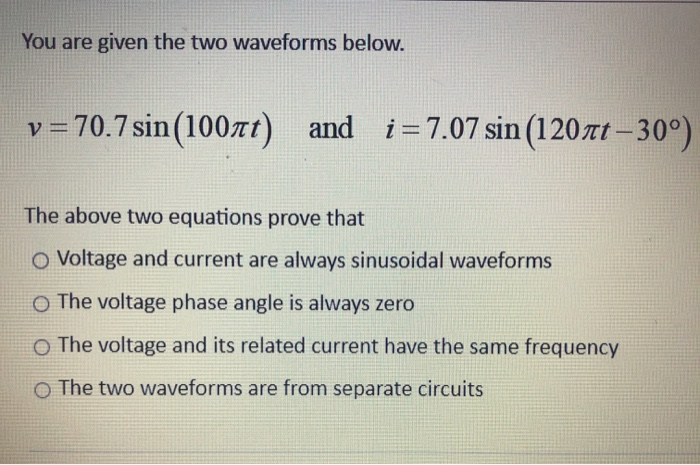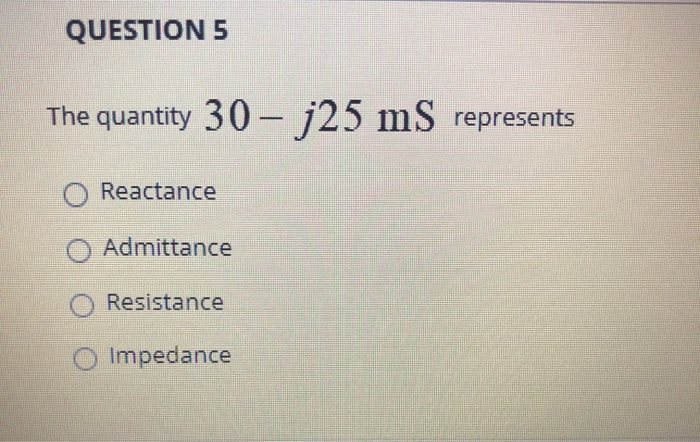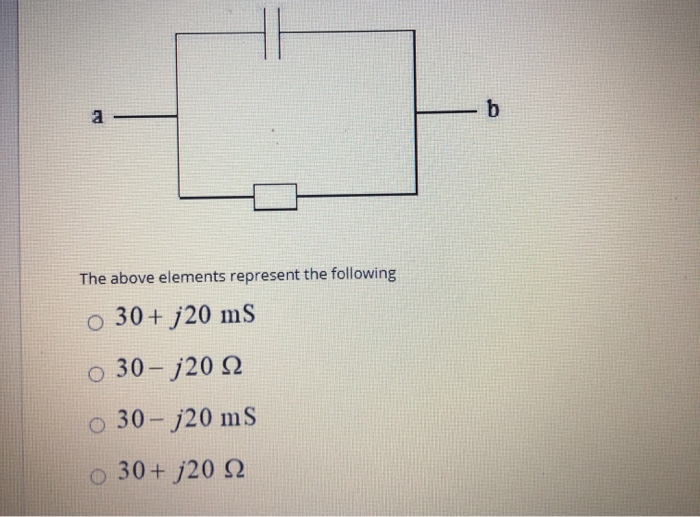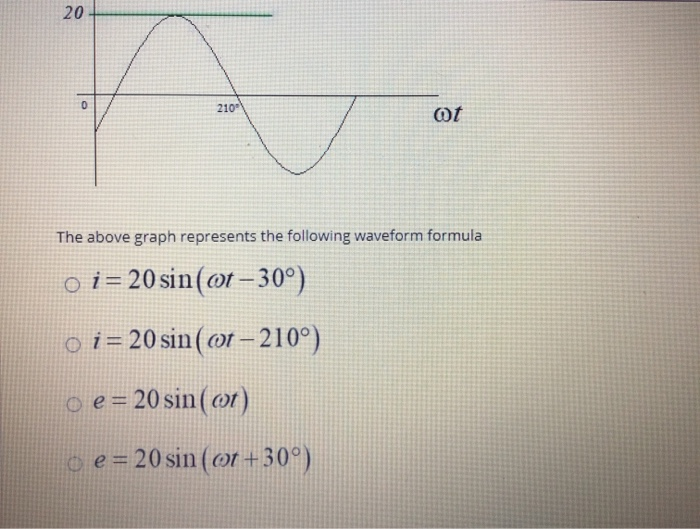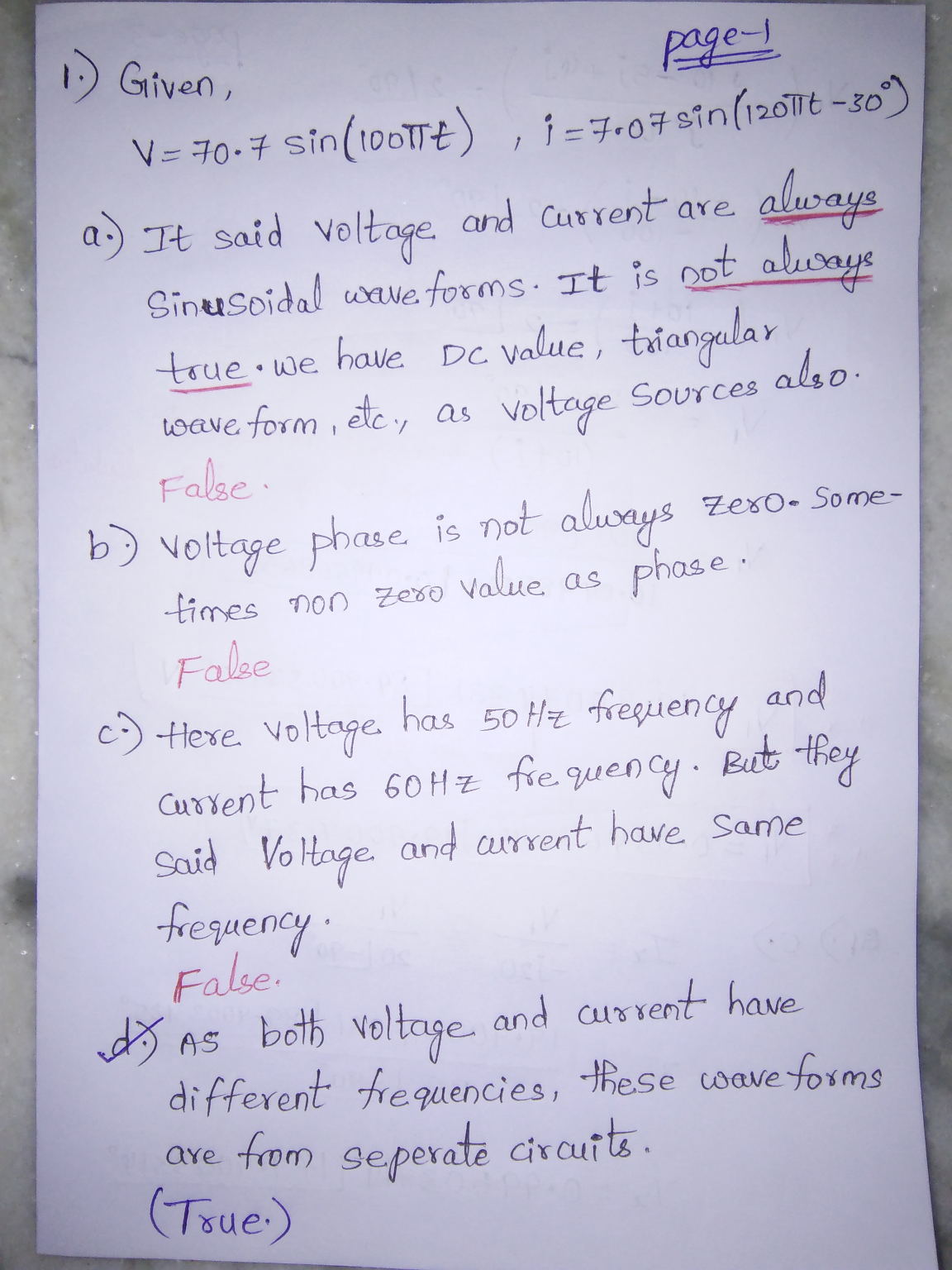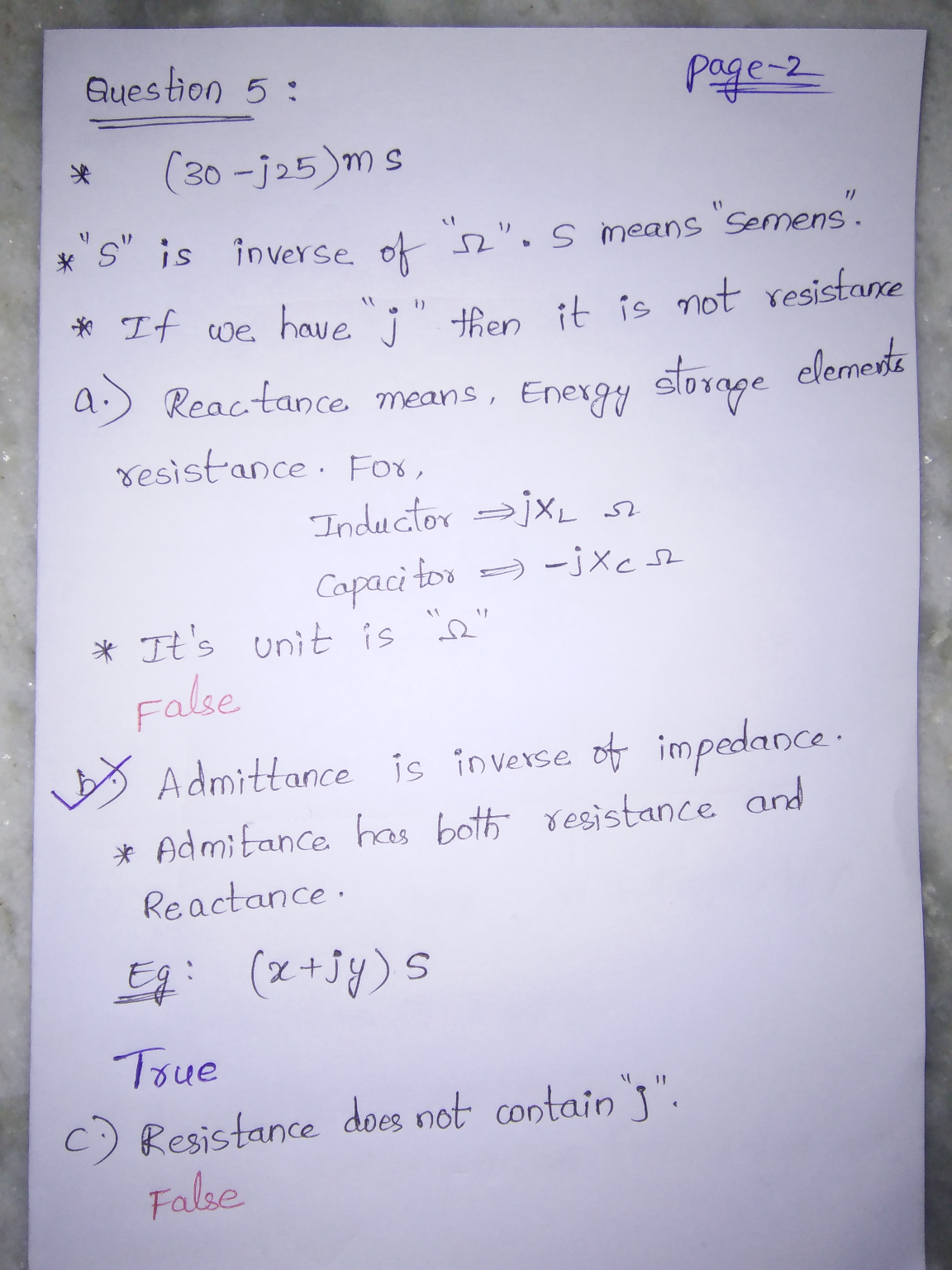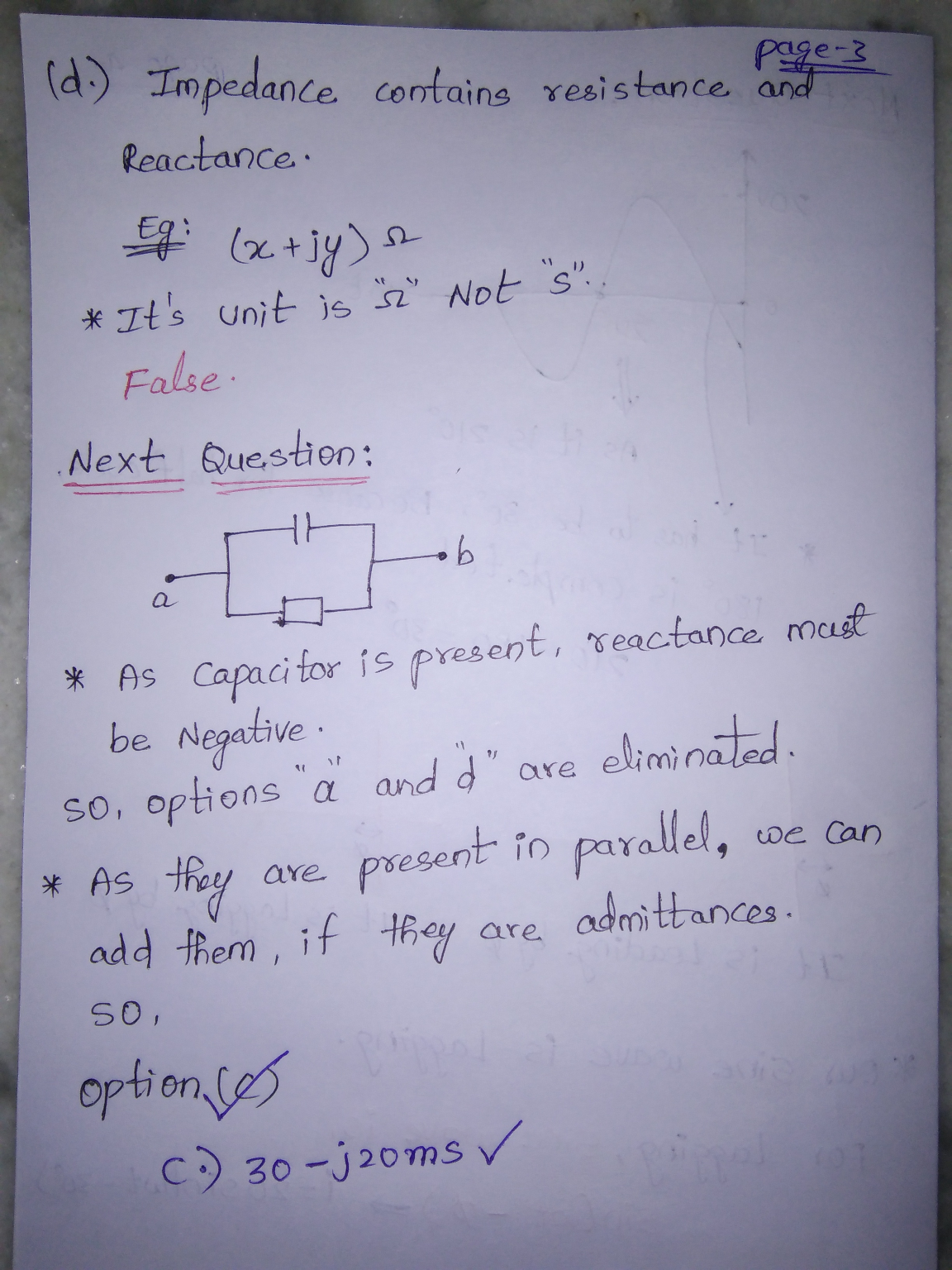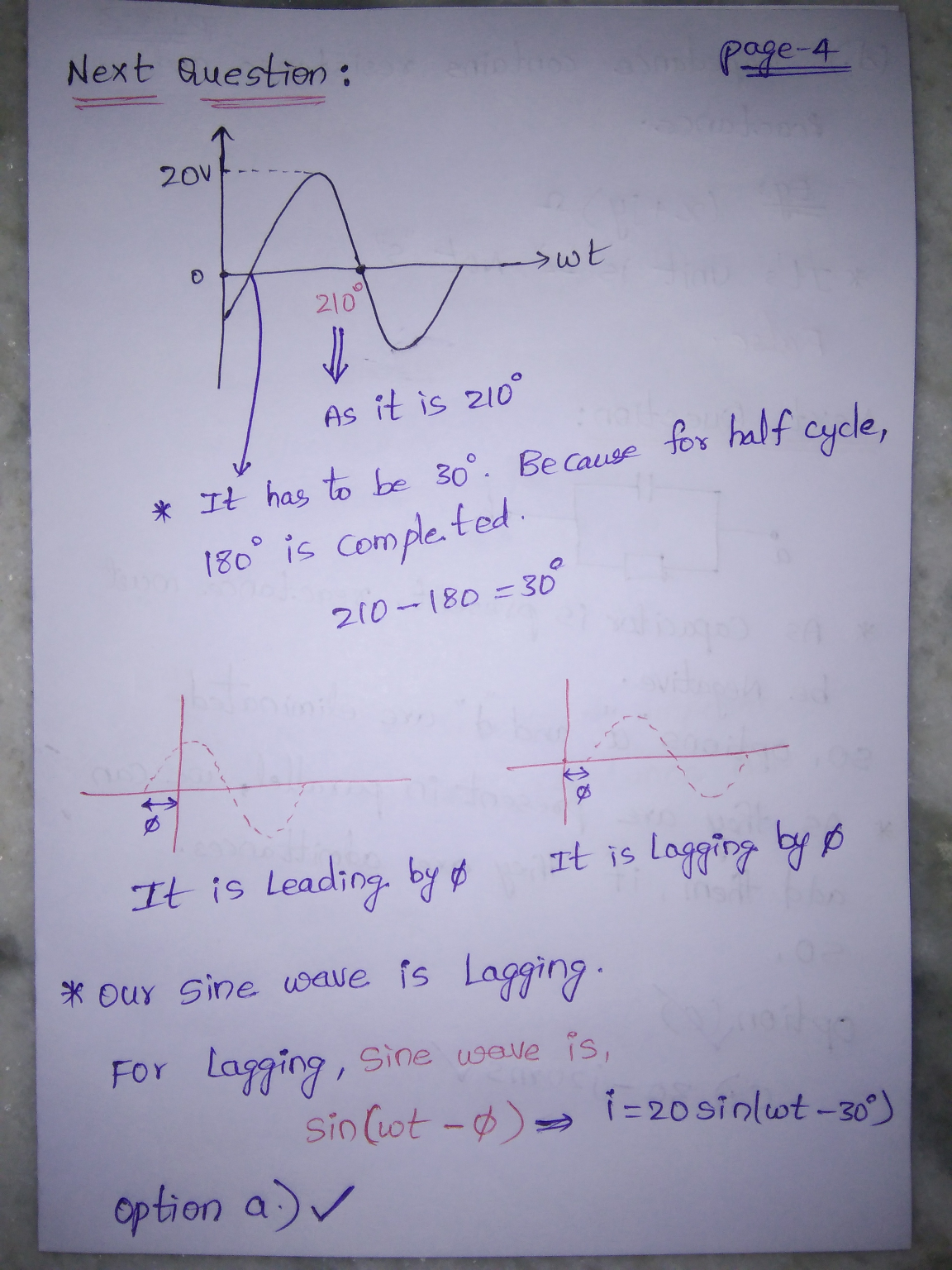#### Earn Coins

Coins can be redeemed for fabulous gifts.

Similar Homework Help Questions
• ### QUESTION 2 You are given the two waveforms below. v = V2 x 20 sin( or...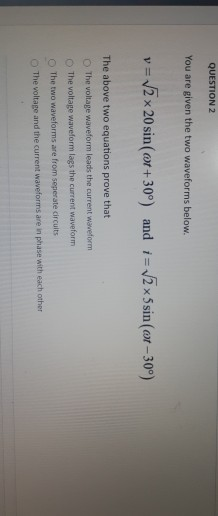QUESTION 2 You are given the two waveforms below. v = V2 x 20 sin( or +30º) and 1 = 2 x 5sin (ct - 30%) The above two equations prove that The voltage waveform leads the current waveform The voltage waveform lags the current waveform The two waveforms are from seperate circuits The voltage and the current waveforms are in phase with each other

• ### PROBLEMS Given in5 cos (400 120%) A, determine the period of he current and the frequency...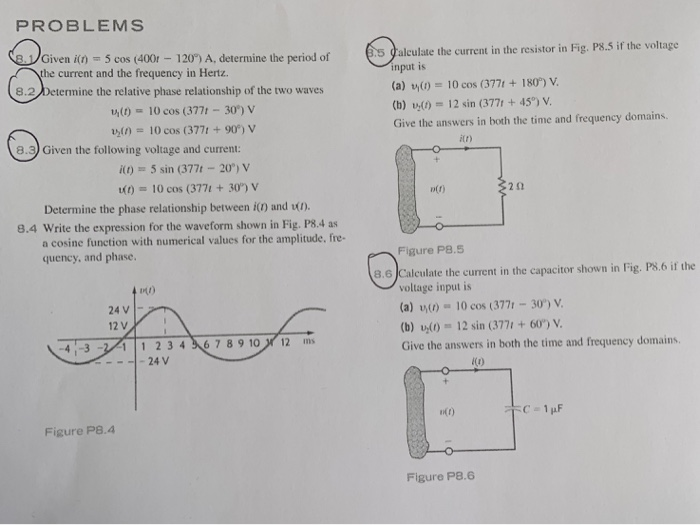PROBLEMS Given in5 cos (400 120%) A, determine the period of he current and the frequency in Hertz. s galculate the current in the resistor in Fig. P8.S if the voltage input is (a) (0)-10 cos (3771 +180°%) V (b) 00 12 sin (377t +45) V Give the answers in both the time and frequency domains 8.2/Determine the relative phase relationship of the two waves ana M(010 cos (377t- 30) v n)10 cos (3771 +90*) v e.9) Given the following...

• ### One of the most important classes of time dependent signals are periodic signals. Periodic signals satisfy tho following signal equations, x(t) X(t) x(t+nt) for n 2,3. The periodic signals to b...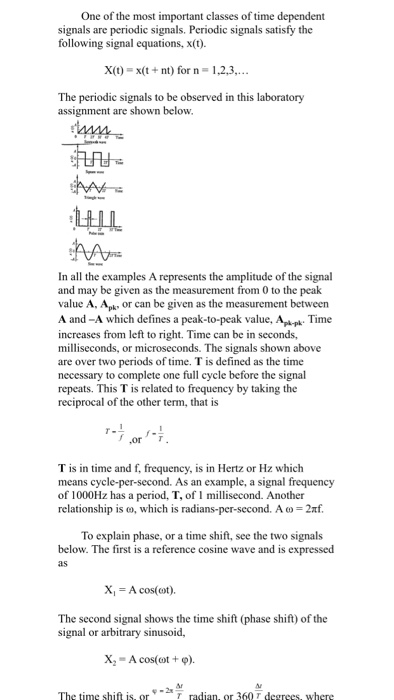One of the most important classes of time dependent signals are periodic signals. Periodic signals satisfy tho following signal equations, x(t) X(t) x(t+nt) for n 2,3. The periodic signals to be observed in this laboratory assignment are shown below. In all the examples A represents the amplitude of the signal and may be given as the measurement from 0 to the peak value A, Apk or can be given as the measurement between A and -A which defines a peak-to-peak...